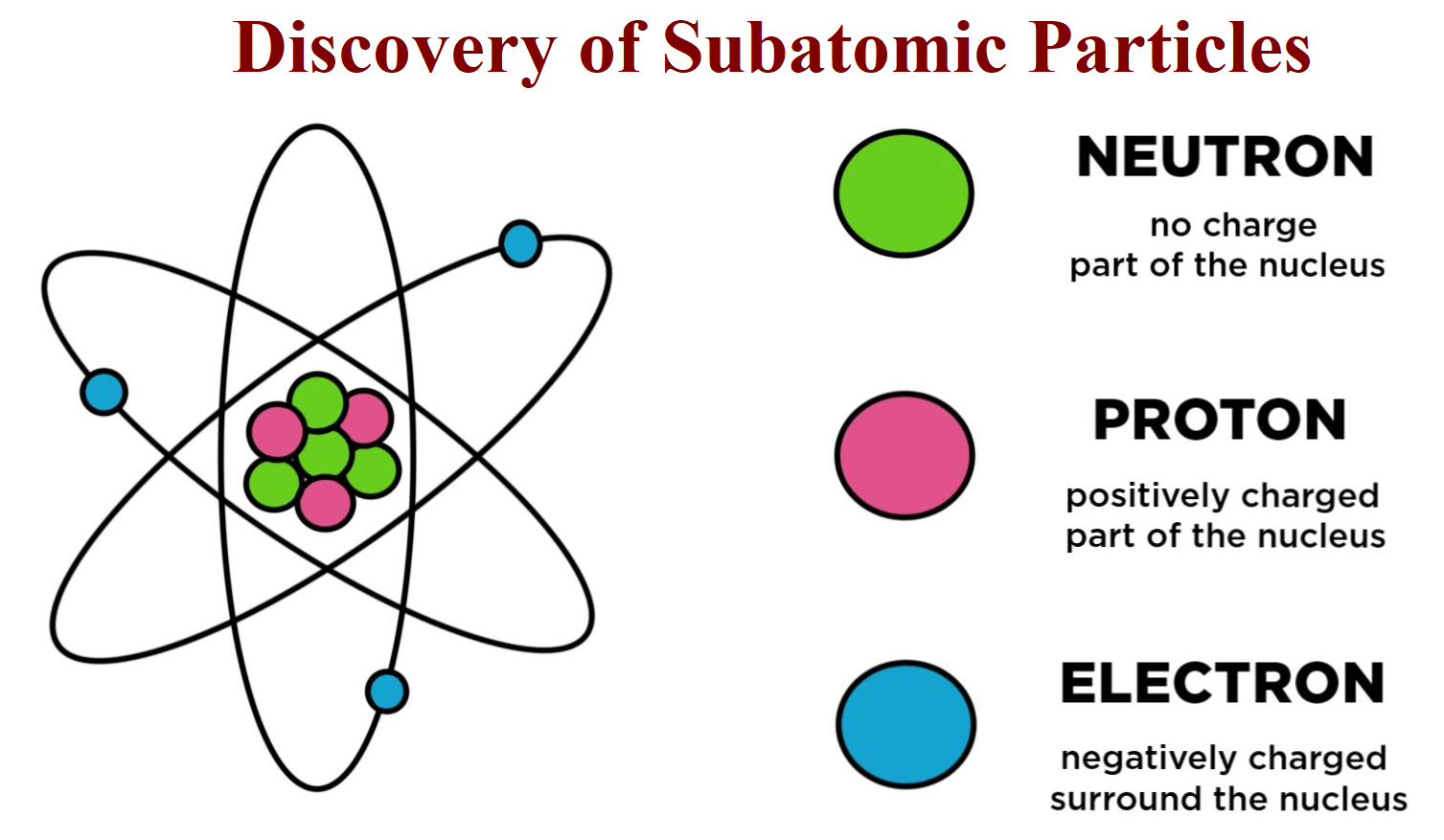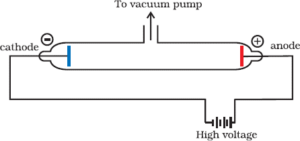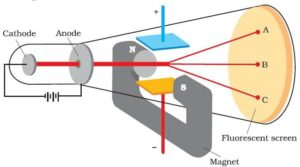# The Discovery of Subatomic ParticlesThe Discovery of Subatomic Particles

This post The Discovery of Subatomic Particles and Atomic Models is all about the subatomic particles their discoveries along with their charges.

STRUCTURE OF ATOM

 Structure of Atom The term atom was introduced by Dalton and is defined as the smallest particle of an element that retains all its properties and identity during a chemical reaction.

• SUB – ATOMIC PARTICLE
• Discovery of Electron

Electron was discovered by J J Thomson by Cathode ray discharge tube experiment. A cathode-ray tube is made of glass containing two thin pieces of metal (called electrodes) sealed in it. The electrical discharge through the gases could be observed only at very low pressures and at very high voltages.When a very high voltage (about 10,000 volts) is applied between the two electrodes, no electric discharge occurs at normal pressure. When the pressure of the gas inside the tube is less than 1 mm of mercury, a dark space appears near the cathode. When the pressure is reduced to 0.01 mm Hg, it fills the whole tube. When the pressure is further reduced (10-4 mm Hg), the electric discharge passes between the electrodes and the tube begins to glow. This is due to the striking of some invisible rays from the cathode. These rays which start from the cathode and move away from it, in straight lines are called cathode rays or cathode ray particles.

These rays can be further checked by making a hole in the anode and coating the tube behind anode with phosphorescent material like zinc sulphide. When these rays strike the zinc sulphide coating, a bright spot on the coating is developed.

• Properties of Cathode Rays
1. The cathode rays start from the cathode and move towards the anode.
2. They are invisible, but their behaviour can be observed with the help of fluorescent or phosphorescent materials.
3. In the absence of an electrical or magnetic field, these rays travel in straight lines.
4. In the presence of the electric or magnetic field, the cathode rays behave similarly to that of negatively charged particles. From this, it is clear that the cathode rays consist of negatively charged particles called electrons.
5. The characteristics of cathode rays (electrons) do not depend upon the material of electrodes and the nature of the gas present in the cathode rays.
6. These rays possess kinetic energy and hence can do mechanical work.
7. They can produce x-rays when incident on metals with high atomic mass.
• Charge to Mass Ratio of Electron:

J.J. Thomson measured the ratio of electrical charge (e) to the mass of the electron (me) by using a cathode ray tube and applying electrical and magnetic field perpendicular to each other as well as to the path of electrons.In the absence of an electric or magnetic field, the cathode rays hit the screen at point B. When only an electric field is applied, the electrons deviate from their path and hit the cathode ray tube at point A. Similarly, when the only a magnetic field is applied, an electron strikes the cathode ray tube at point C. By carefully balancing the electrical and magnetic field strength, it is possible to bring back the electron beam to point B. From the strength of the electric and magnetic field, Thomson was able to calculate the value of e/me as:
e/me = 1.758 × 1011 C kg–1 Where me is the mass of the electron in kg and e is the magnitude of the charge on the electron in coulomb (C).

• Charge on the Electron (e):

R.A. Millikan determined the charge on the electrons by a method known as the ‘oil drop experiment’. He found that the charge on the electron to be 1.6022 × 10–19 C.

Mass of Electron (me):
The mass of the electron (me) was determined as follows:
Mass of electron (me) = e / e/me = 1.6022 × 10-19/1.758 × 1011
Mass of Electron = 9.1 × 10-31 kg

• Discovery of Protons:

E. Goldstein modified the discharge tube experiment by perforated (with small holes) the cathode. After evacuating the tube and on applying high voltage, he found that some rays were emitting behind the cathode and moving in the opposite direction of cathode rays. These rays deflect to the negative plate of the electric field. So they carry a positive charge and were called anode rays or canal rays.

• Discovery of Neutrons:

Neutrons were discovered by James Chadwick by bombarding a thin sheet of beryllium by α-particles.
4Be9 + 2He46C12 + 0n1
They are electrically neutral particles having mass slightly greater than that of the protons.

Characteristics of Sub – Atomic Particles:

 Sub Atomic Particle Symbol Discoverer Absolute Charge (in Coulomb) Relative Charge Mass In kg Electron e J.J. Thomson -1.6022 × 10-19 -1 9.01×10-31 Proton p E. Goldstein +1.6022 ×10-19 +1 1.6726×10-27 Neutron n James Chadwick 0 0 1.675×10-27

This is all about various experiments involved in The Discovery of Subatomic Particles.

• Atomic Number: It is the number of protons present in the nucleus or the number of electrons present outside the nucleus.

It is denoted by the symbol ‘Z’.
Atomic number (Z) = nuclear charge or number of protons (p)
= number of electrons (e)

• Mass Number: It is the total number of protons and neutrons in atom. Or, it is the total number of nucleons in an atom.

It is denoted by ‘A’.
i.e. Mass number (A) = no. of protons (p) + no. of neutrons (n) or, A = p + n
By knowing the atomic number and mass number, we can calculate the number of neutrons as:
n = A – Z If an element X has the atomic number Z and the mass number A, it is denoted as:  ZXA